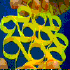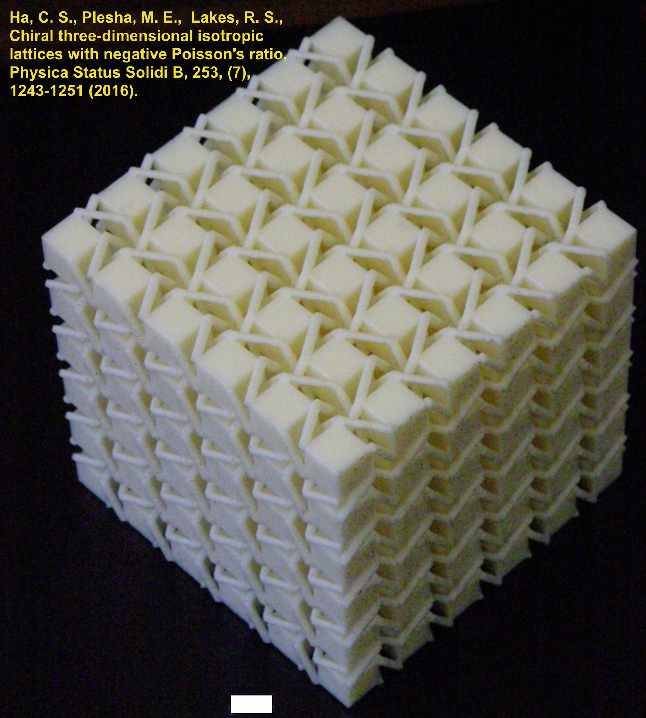# Chiral Cosserat elasticity

## Rod Lakes

Chiral materials do not have a center of inversion symmetry. Left and right handed versions of the material are distinct. Chiral materials are also called hemitropic or noncentrosymmetric. Some material properties such as piezoelectricity and pyroelectricity can only occur in chiral materials. Classical elasticity makes no distinction between chiral and non-chiral materials because the elastic modulus tensor is fourth rank. By contrast, generalized continuum theories such as Cosserat elasticity do make such a distinction.
Curiously the subject of chiral elasticity has recently become popular. Our research in this area is summarized below: the first prediction of stretch twist or squeeze twist coupling in an elastic solid represented as a continuum. We have performed the first experimental demonstrations of chiral elastic behavior.

Rod Lakes and Robert Benedict, Noncentrosymmetry in micropolar elasticity, International Journal of Engineering Science, 20 (10), 1161-1167, (1982).
A solid which is isotropic with respect to coordinate rotations but not with respect to inversions is called noncentrosymmetric, acentric, hemitropic, or chiral. Chirality has no effect upon the classical elastic modulus tensor. In Cosserat elasticity, chirality (hemitropy) has an effect. A chiral Cosserat solid has three new elastic constants in addition to the six considered in the fully isotropic micropolar solid. The chiral micropolar solid is predicted to undergo torsional deformation when subjected to tensile load. Thus chiral solids have different mechanical behavior from solids with a center of symmetry, as allowed by the more general Cosserat elastic theory. The theory predicts coupling between stretching and twisting.Prall, D. and Lakes, R. S., Properties of a chiral honeycomb with a Poisson's ratio -1", Int. J. of Mechanical Sciences, 39, 305-314, (1997).
A theoretical and experimental investigation is conducted of a two-dimensionally chiral honeycomb. The honeycomb exhibits a Poisson's ratio of -1 for deformations in-plane. This Poisson's ratio is maintained over a significant range of strain, in contrast to the variation with strain seen in known negative Poisson's ratio materials.
This is the first two-dimensional metamaterial that is chiral; we did not call it by such a name.

Lakes, R. S., Third-rank piezoelectricity in isotropic chiral solids, Appl. Phys. Lett., 106, 212905, May (2015). The highest symmetry in which piezoelectricity was thought to occur is cubic. Here, it is shown that third rank piezoelectricity can occur in isotropic chiral solids. Polarization is coupled via an isotropic third rank tensor to the antisymmetric part of the stress. Asymmetric stress can occur if balanced by moments distributed over area or volume. Such moments occur in heterogeneous solids, in which there exists a characteristic length associated with the microstructure: the Cosserat or micropolar solids. Effects associated with nonzero structure size are predicted, including radial polarization in response to torsion. These effects do not occur in gradient type flexoelectric materials; they are governed by a different tensorial rank and symmetry. DOI: 10.1063/1.4921846 journal link       Get preprint pdf

Ha, C. S., Plesha, M. E., Lakes, R. S., "Chiral three dimensional lattices with tunable Poisson's ratio, Smart Materials and Structures, 25, 054005 (6pp) (2016). Chiral three dimensional cubic lattices are developed with rigid cubical nodules and analyzed via finite element analysis. The lattices exhibit geometry dependent Poisson's ratio that can be tuned to negative values. Poisson's ratio tends to zero as the cubes become further apart. The lattices exhibit stretch twist coupling. Such coupling cannot occur in a classical elastic continuum but it can occur in a chiral Cosserat solid.
preprint pdfHa, C. S., Plesha, M. E., Lakes, R. S., Chiral three-dimensional isotropic lattices with negative Poisson's ratio, Physica Status Solidi B, 253, (7), 1243-1251 (2016). DOI: 10.1002/pssb.201600055
Chiral three-dimensional isotropic cubic lattices with rigid cubical nodules and multiple deformable ribs are developed and analyzed via finite element analysis. The lattices exhibit geometry dependent Poisson's ratio that can be tuned to negative values. Poisson's ratio decreases from positive to negative values as the number of cells increases. Isotropy is obtained by adjustment of aspect ratio. The lattices exhibit significant size effects. Such a phenomenon cannot occur in a classical elastic continuum but it can occur in a Cosserat solid. The material exhibits squeeze-twist coupling and stretch-twist coupling. Coupling of this type cannot occur in classical elasticity. Lattices with this property are made by 3D printing.
This is the first three-dimensional metamaterial that is chiral; we did not call it by such a name.
preprint pdf

D. Reasa and R. S. Lakes, "Cosserat effects in achiral and chiral cubic lattices", Journal of Applied Mechanics (JAM), 86, 111009-1, 6 pages Nov. (2019).
Cubic 3D lattices were designed, made by 3D printing, and studied experimentally. One lattice was simple cubic with ribs of diameter 1/5 the cell size. The second lattice was chiral, with spiral ribs. The chiral lattice, but not the achiral lattice, exhibited squeeze-twist coupling with size effects. Squeeze-twist coupling cannot occur in a classically elastic solid but is anticipated by theory in a Cosserat solid. Both lattices exhibited size effects in bending and torsion.
https://doi.org/10.1115/1.4044047 https://doi.org/10.1115/1.4044047

J. Li, C. S. Ha, R. S. Lakes, "Observation of squeeze twist coupling in a chiral three-dimensional isotropic lattice", Physica Status Solidi B Volume 257, Issue10, 1900140, October (2020).
A chiral 3D lattice was designed, made by 3D printing, and studied experimentally. The lattice exhibited squeeze-twist coupling and a Poisson's ratio near zero. Squeeze-twist coupling does not occur in classical elasticity which makes no provision for chirality. By contrast, chiral effects are allowed in Cosserat elasticity. An experimental squeeze-twist coupling strain ratio on the order of unity and a Poisson's ratio near zero are in reasonable agreement with prior finite element analysis of a lattice with similar structure, for which negative Poisson's ratio is anticipated for a sufficient number of cells.